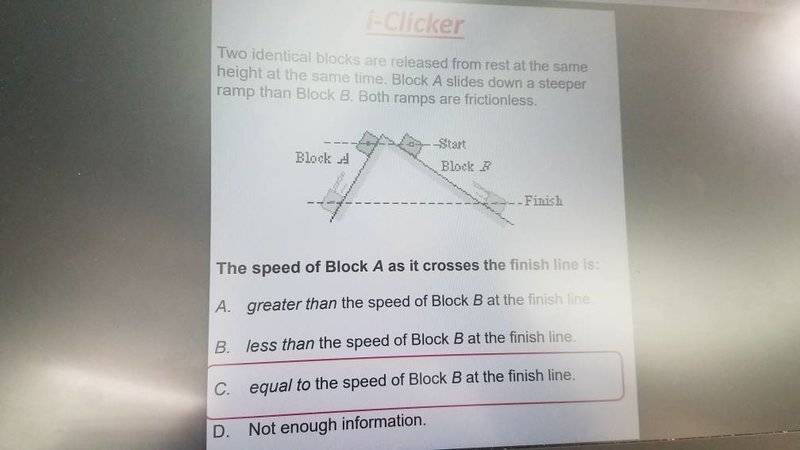# Work and Kinetic Energy

• Lori

#### Lori

I have the problem below.

I know that net work is equal to the change in kinetic energy which is Wnet= 0.5mv^2-0.5mvi^2.

I also know that the work by force of gravity is the same for both objects. I don't understand why the objects would be the same speed at the finish line.#### Attachments

The speed will be the same. This does not mean that they will reach the finish line at the same time, block B has to travel longer.

The speed will be the same. This does not mean that they will reach the finish line at the same time, block B has to travel longer.
Can you explain why this would be the case? Would it be because net work is the same for both objects? Because if that's the case, then KE has to be the same and so the final velocities are the same.

However. I wouldn't know why new work is the same for both objects.

Would it be because net work is the same for both objects? Because if that's the case, then KE has to be the same and so the final velocities are the same.
Yes.

I wouldn't know why new work is the same for both objects.
You said it yourself in the first post:
I also know that the work by force of gravity is the same for both objects.

•Lori
Yes.

You said it yourself in the first post:
Ahh yeah. I wasn't sure if force of gravity was the only work done on object but it makes sense since the ramp is frictionless and gravity is the only force applied.

Ahh yeah. I wasn't sure if force of gravity was the only work done on object but it makes sense since the ramp is frictionless and gravity is the only force applied.
Gravity is not the only force. There is also a normal force from the ramp. Can you figure out why it does no work?

Gravity is not the only force. There is also a normal force from the ramp. Can you figure out why it does no work?
Oh . Well the component in the y direction of gravity balances out normal force. So the normal force doesn't do any work.

Oh . Well the component in the y direction of gravity balances out normal force. So the normal force doesn't do any work.
It is not really a matter of force equilibrium. Whether a force does work or not depends on the force's component parallel to the displacement.

It is not really a matter of force equilibrium. Whether a force does work or not depends on the force's component parallel to the displacement.
Oh right! I was talking about net work. So the work that normal force does is 0